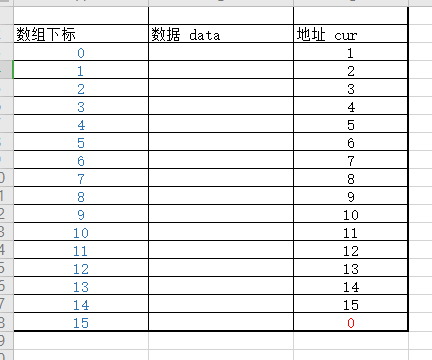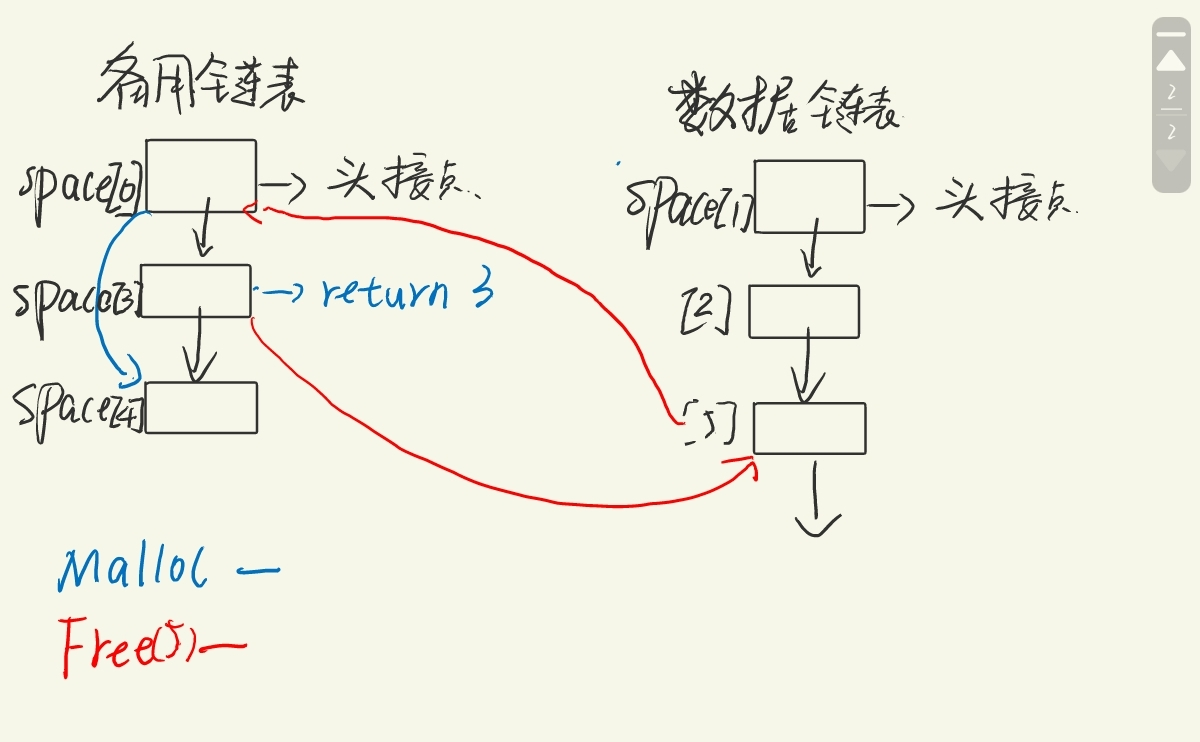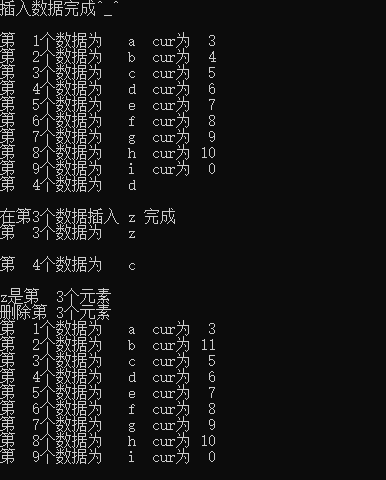## 静态链表

typedef struct
{
ElemType data;
int cur;//其实可以把它看着指针，指针也不少一个数吗？


cur: 就是下一个结点的数组下标，其实可以把它看着指针，指针也不少一个数吗？

### 算法解析

• cur = 0，表示结尾
• cur =MaxSize ，表示没有使用该段空间

#### 代码

##### InitSpace _SL

void InitSpace _SL(SLinkList &space)
{
for(i=0;i<MaxSize-1;i++) //数组下标从0开始，只需要循环到 MaxSize-2
space[i].cur=i+1;
space[MaxSize - 1]==0;//0表示NULL ,space[MaxSize - 1]也就是 备用链表的尾结点
}##### Malloc_SL

int Malloc_SL(SLinkList &space)
{
i=space.cur;//备用链表的第一个结点取出
if(space.cur)//如果 space.cur==0 说明备用链表被使用完了，分配空间失败
space.cur=space[i].cur;//将取出的结点从备用链表中删除
return i;
}

##### Free_SL

Free_SL(SLinkList &space ,int k)
{
space[k].cur=space.cur;sapce.cur=k;
}##### difference

void difference(SLinkList &space,int &S)
{
InitSpace_SL(space);   //初始化静态链表（创建备用链表）
S=Malloc_SL(space);    //生成数据链的头结点
r=S;                   //r为当前A集合链表的表尾
scanf(m,n);           //输入数据链表的大小 m :A  n: B
//生成链表A,并填入数据
for(j=1;j<=m;++j)
{
i=Malloc_SL(space);
Scanf(space[i].data);//输入数据
space[r].cur=i;      //插入数据到数据链表
r=i;                 //r为当前A集合链表的表尾
}
space[r].cur=0;          //数据链尾结点为NULL(0)
for(j=1;j<=n;j++)
{
scanf(b);
p=S;  //p存储着要删除结点前驱结点
k=space[S].cur; //k指向集合A元素的下一个结点
//遍历A集合 数据b是否也在A中（是否重复）
while(k!=space[r].cur&&space[k].data!=b)
// k!=space[r].cur 到达存储A集合链表的表尾 space[k].data!=b b不在元素 A
{
p=k;//p存储着要删除结点前驱结点
k=space[k].cur;//遍历下一个结点
}
if(k==Space[r].cur)
//数据b没有重复，加入数据b
//数据b插入到 r结点之后
{
i=Malloc_SL(space);
space[i].data=b;
space[i].cur=space[r].cur;
space[r].cur=i;
}
else//数据B重复了
{
space[p].cur=space[k].cur;   //此时K删除点
if(r==k) //如果删除的是r结点，需要改r的指向
r=p;
}
}
}


### 静态链表代码

#include<stdio.h>

/**

1. 专门设置一个数值例如 cur=-1 表示改节点没有被使用

2.  在静态链表中 存着两个链表

**/

#define MaxSize 100
typedef struct
{
char data;
int cur;

void test()
{
char e;
//插入数据测试
for (int i=1; i < 10; i++)
{
}
printf("插入数据完成^_^\n \n");
int i=L.cur;
int j=1;
while (i)
{
printf("第%3d个数据为 %3c  cur为%3d\n", j, L[i].data,L[i].cur );
i = L[i].cur;
j++;
}
//获取修改数据测试
printf("第  4个数据为 %3c\n\n", e);
printf("在第3个数据插入 z 完成\n");
printf("第  3个数据为 %3c\n\n", e);
printf("第  4个数据为 %3c\n\n", e);
//删除数据测试
printf("删除第 3个元素\n");
i = L.cur;
j = 1;
while (i)
{
printf("第%3d个数据为 %3c  cur为%3d\n", j, L[i].data, L[i].cur);
i = L[i].cur;
j++;
}
getchar();
}
int main()
{
test();
return 0;
}

/*

*/
{
//备用连的头结点 L，创建备用链表
for (int i = 2; i < MaxSize - 1; i++) //遍历到MaxSize -2即可
L[i].cur = i+1;
//第一个结点从2开始
L.cur = 2;
//备用链表表尾
L[MaxSize-1].cur = 0;

//创建数据链表的头结点  L
L.cur = 0;
return true;
}
/*

0表示链表已满
*/
{
int i = L.cur;//从备用链表取出的结点
if (L.cur)//如果备用链表没有满
L.cur = L[i].cur;//该结点从备用链表中删除
return i;
}

{
//将k结点和后面一个结点交换数据
int p = L[k].cur;
L[k].data = L[p].data;
L[k].cur= L[p].cur;
//删除结点p
L[p].cur = L.cur; L.cur = p;
return true;
}

{
if (i<1 || i>MaxSize - 2)
return false;
int p = L.cur;
int j = 1;//计数器
//遍历数据链表
while (p && j < i)
{
p = L[p].cur;
j++;
}
if (!p && j > i)//i<0或者第i个数据不存在
return false;
k = p;
return true;
}

{
if (k<1 || k >= MaxSize) //结点不存在
return false;
int p = MallocNode(L);
if (!p)//如果分配失败
return false;
L[p].data = e;
L[p].cur = L[k].cur;
L[k].cur = p;
return false;
}

{
int k;
return false;
}

{
int k = L.cur;
int i = 1;
while (k && L[k].data != e)
{
k = L[k].cur;
i++;
}
if (k == 0)//如果e不存在
i = 0;
return i;
}

{
int k;
return false;
e = L[k].data;
return false;
}
{
int k;
return false;
e = L[k].data;
return false;
}Q.E.D.